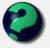Discussion about math, puzzles, games and fun.   Useful symbols: ÷ × ½ √ ∞ ≠ ≤ ≥ ≈ ⇒ ± ∈ Δ θ ∴ ∑ ∫  π  -¹ ² ³ °

You are not logged in.

## #1 2007-11-15 09:56:53

freddogtgj
Member
Registered: 2006-12-02
Posts: 54

### Linear Algebra Help Needed!

Hi this is the question that's troubling me:

Show the following

(a) If

is a spanning set for a vector space V and v is any vector in V, then
are linearly dependent.

(b) If

are linearly independent vectors in a vector space V, then
cannot span V.

Offline

## #2 2007-11-15 10:48:30

mathsyperson
ModeratorRegistered: 2005-06-22
Posts: 4,900

### Re: Linear Algebra Help Needed!

By definition of a spanning set, any vector in V can be made by a linear combination of the vectors v_1, ..., v_n, including v.

That is, there exist scalars α_1, ..., α_n such that α_1v_1 + α_2v_2 + ... + α_nv_n = v.

Rearranging gives that α_1v_1 + α_2v_2 + ... + α_nv_n + (-1)v = 0.
That means that there is a linear combination of the vectors v_1, ..., v_n, v (with at least one of the scalars not zero) that adds to 0. Therefore, the set is linearly dependent.

---

Assume that v_2, ..., v_n span V. Then, by the previous result, v_1, v_2, ..., v_n are a linearly dependent set of vectors. Contradiction; therefore v_2, ..., v_n do not span V.

Why did the vector cross the road?
It wanted to be normal.

Offline